February 28, 2017

## Forecast Library

To compute an ACF or PACF, use the `forecast` package.

Install via Tools > Install Packages… menu.

`library("forecast")`
`Loading required package: zoo`
`Attaching package: 'zoo'`
```The following objects are masked from 'package:base':

as.Date, as.Date.numeric```
`Loading required package: timeDate`
`Loading required package: methods`
`This is forecast 7.3 `

## ACF for White Noise

```n <- 10000; time <- 1:n
wn <- rt(n, df=3)
Acf(wn)```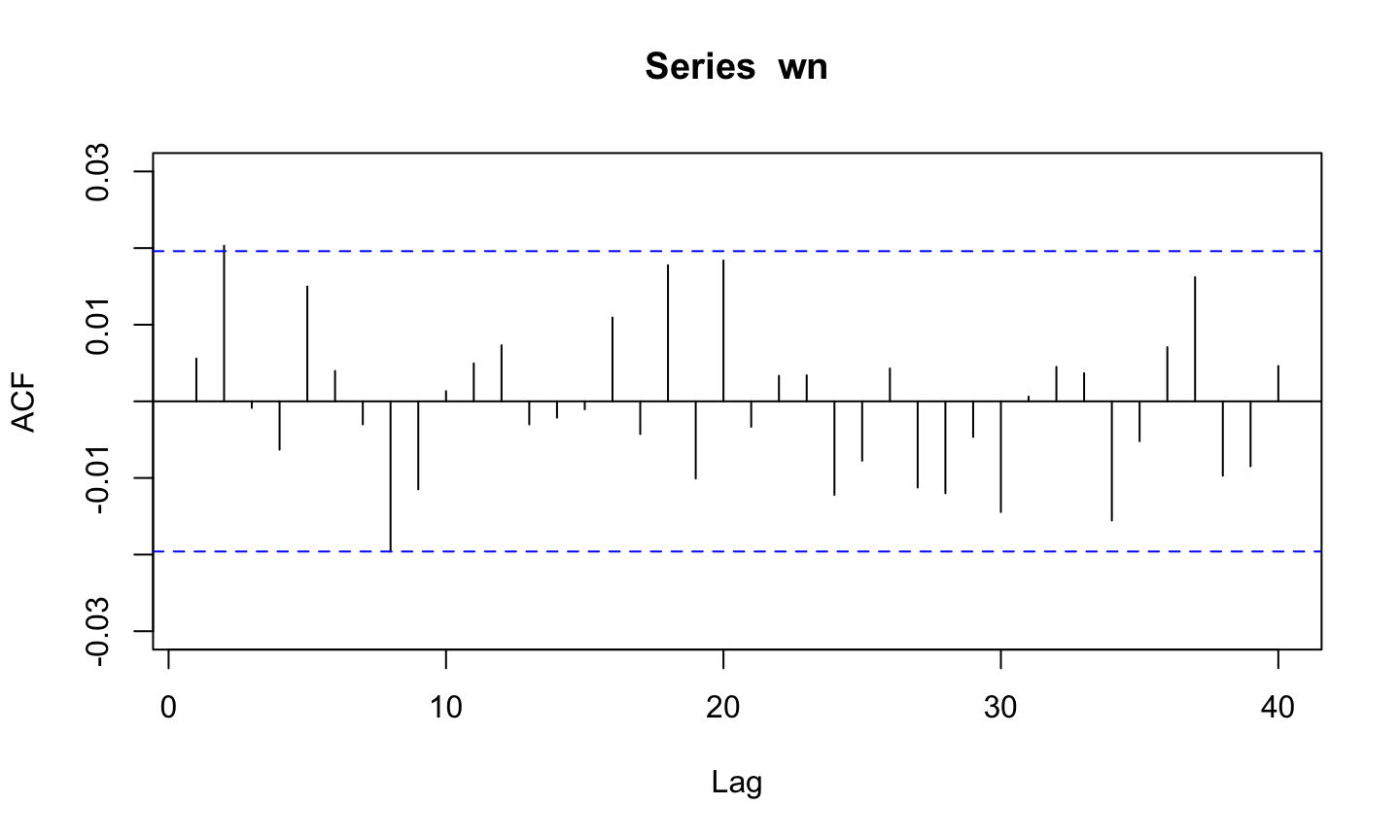## ACF for MA(1) and MA(2)

```ma1 <- filter(wn, c(1, 0.25), method="convolution", sides=1)
ma2 <- filter(wn, c(1, 0.1, 0.25), method="convolution", sides=1)
par(mfrow=c(1,2))
Acf(ma1); Acf(ma2)```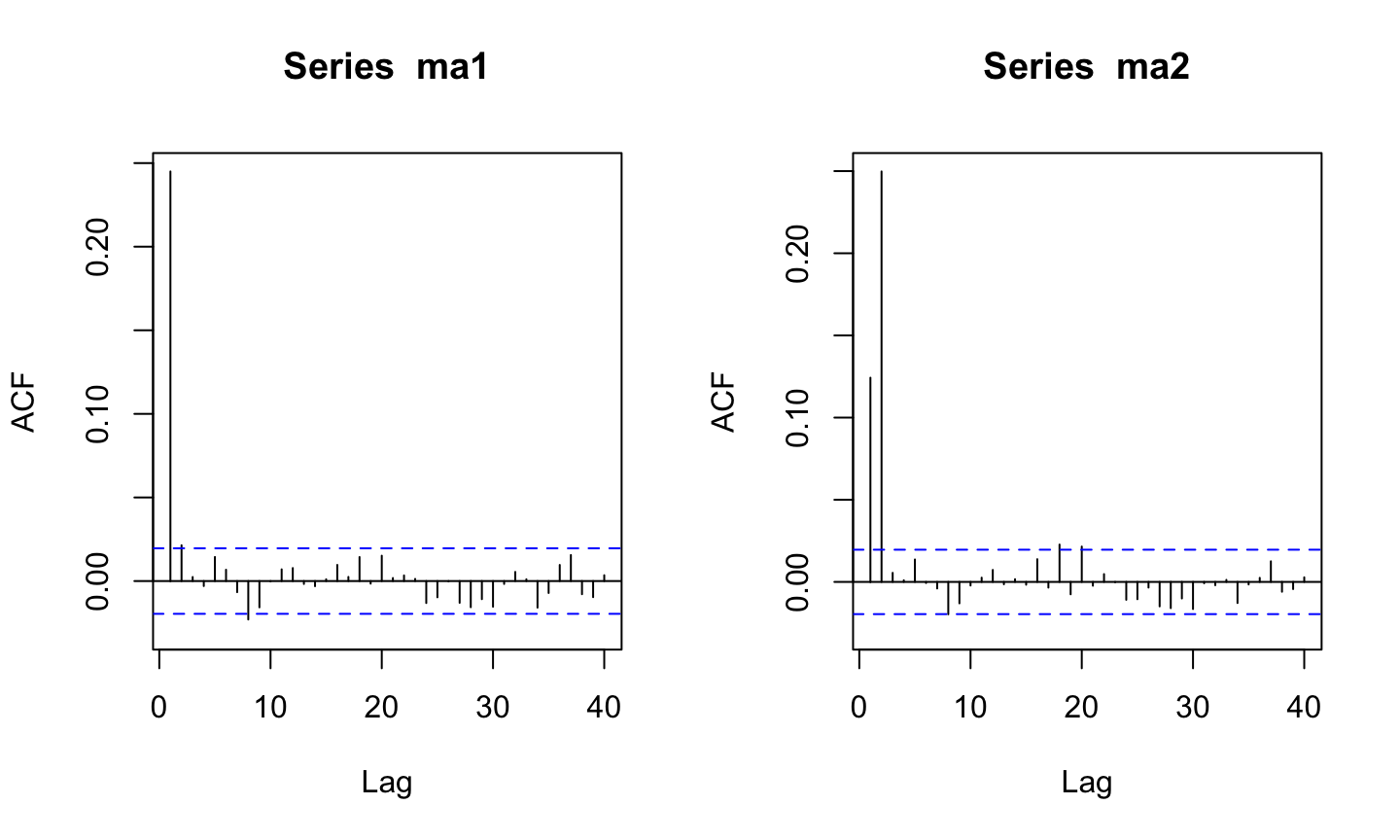## ACF for AR(1) and AR(2)

```ar1 <- filter(wn, 0.5, method="recursive")
ar2 <- filter(wn, c(0.4, -0.2), method="recursive")
par(mfrow=c(1,2))
Acf(ar1); Acf(ar2)```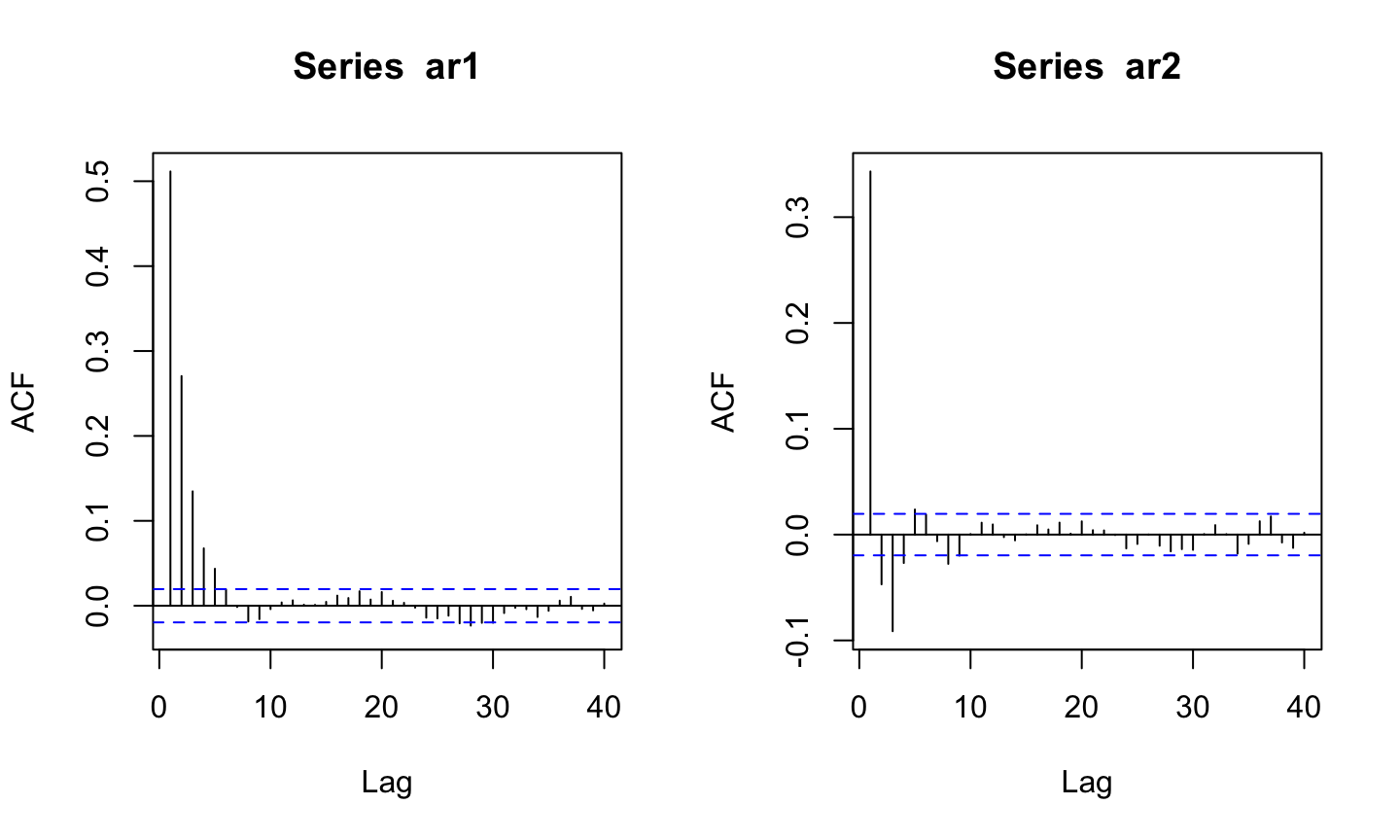## PACF for White Noise

`Pacf(wn)`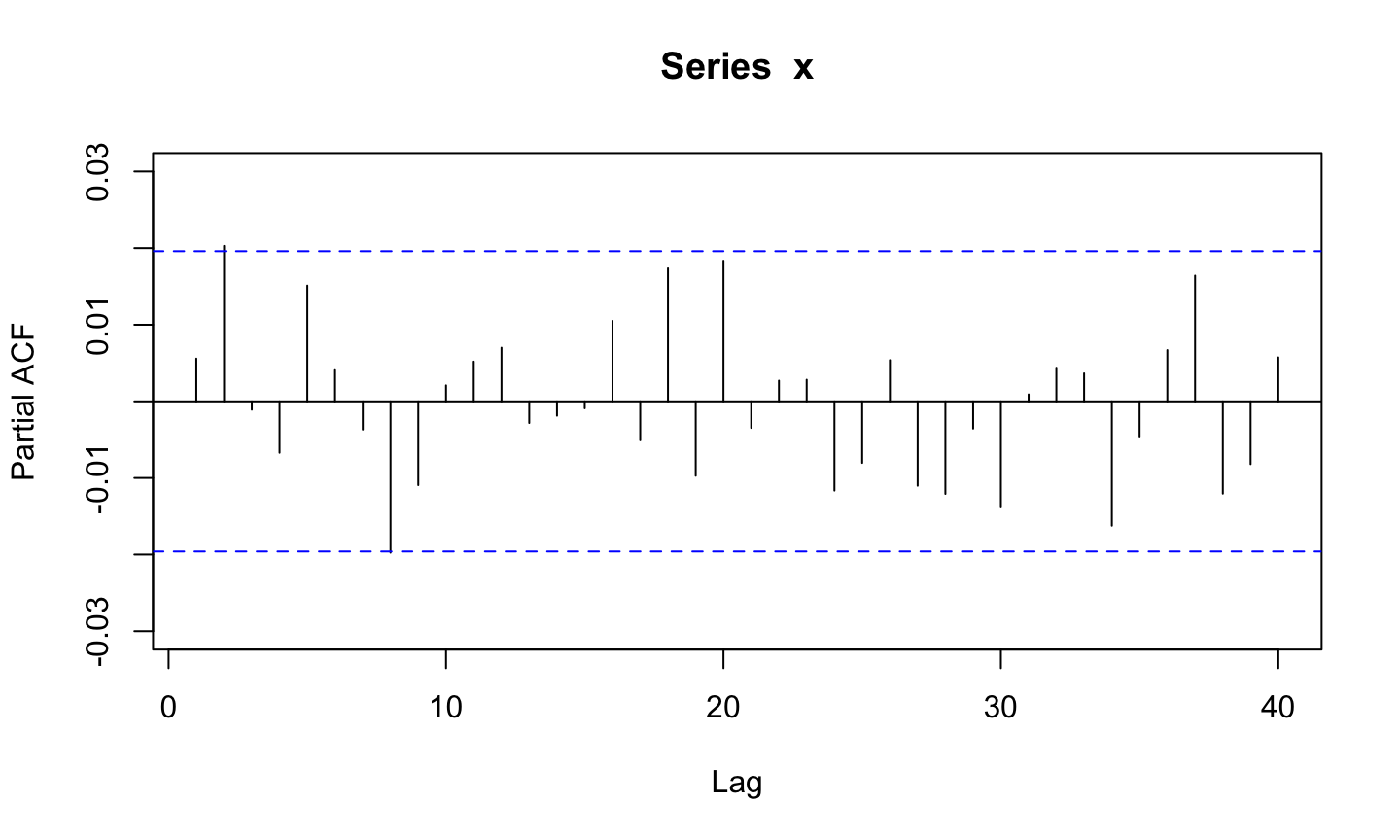## PACF for AR(1) and AR(2)

```par(mfrow=c(1,2))
Pacf(ar1)
Pacf(ar2)```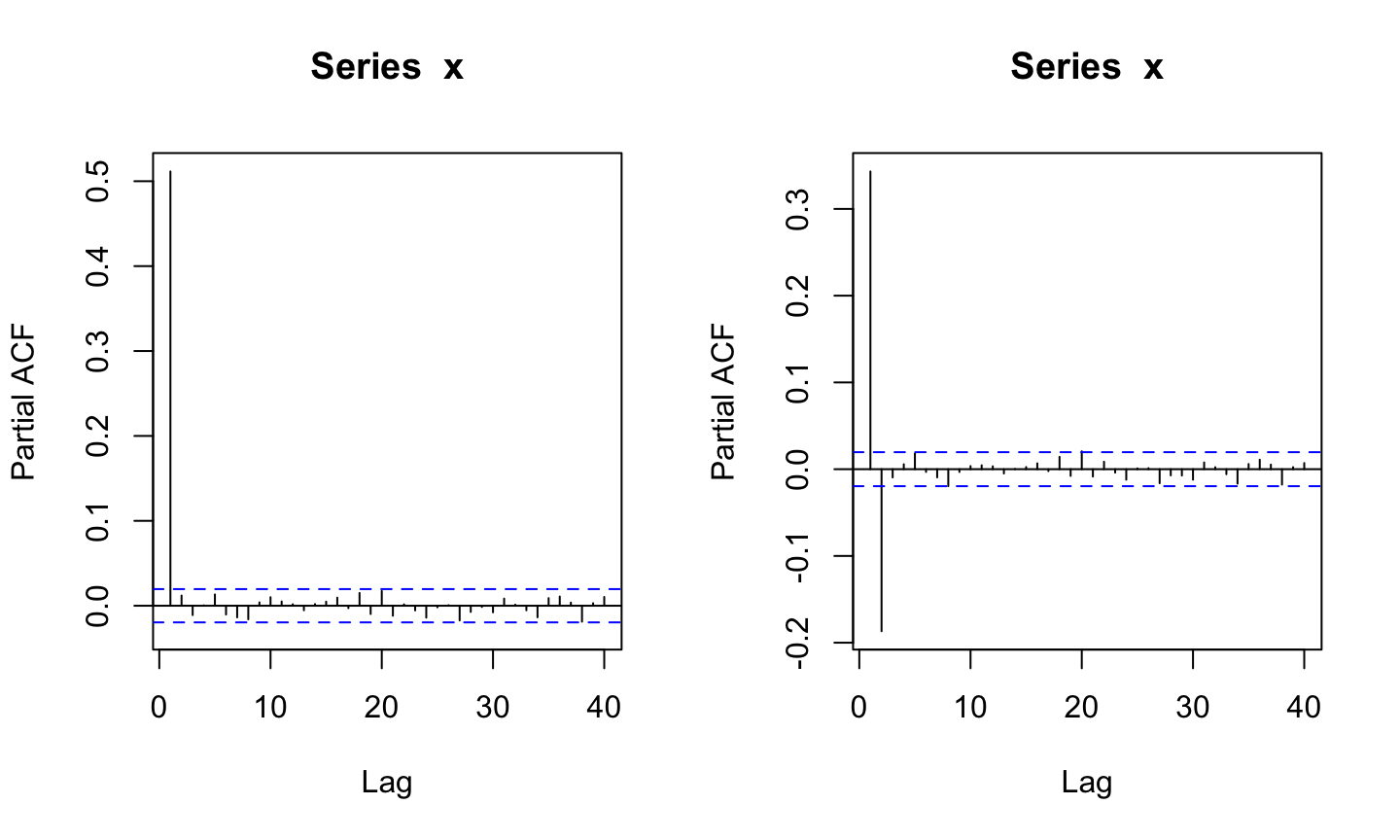## PACF for MA(1) and MA(2)

```par(mfrow=c(1,2))
Pacf(ma1)
Pacf(ma2)```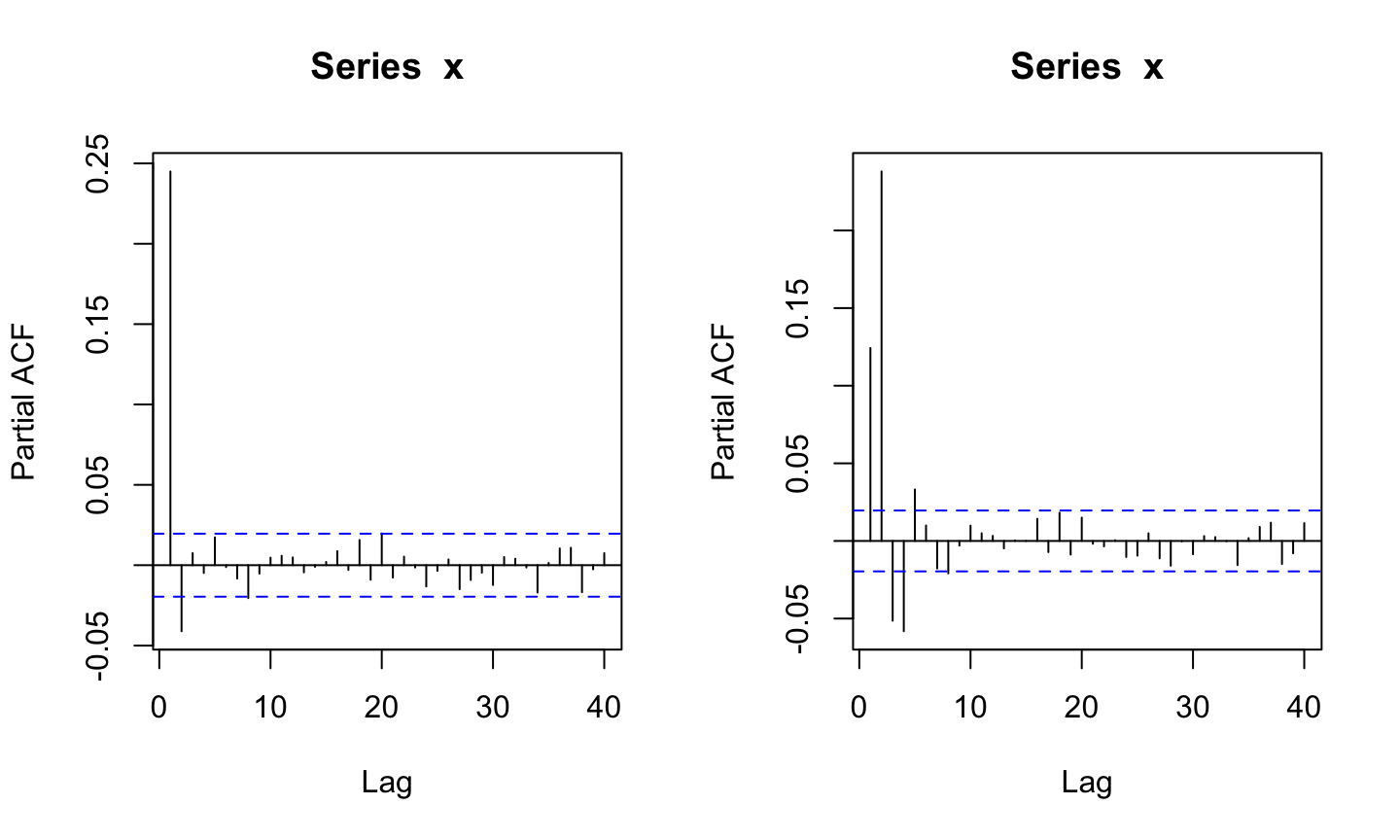## Over-Differencing

```par(mfrow=c(1,2))
Acf(wn); Acf(diff(wn))```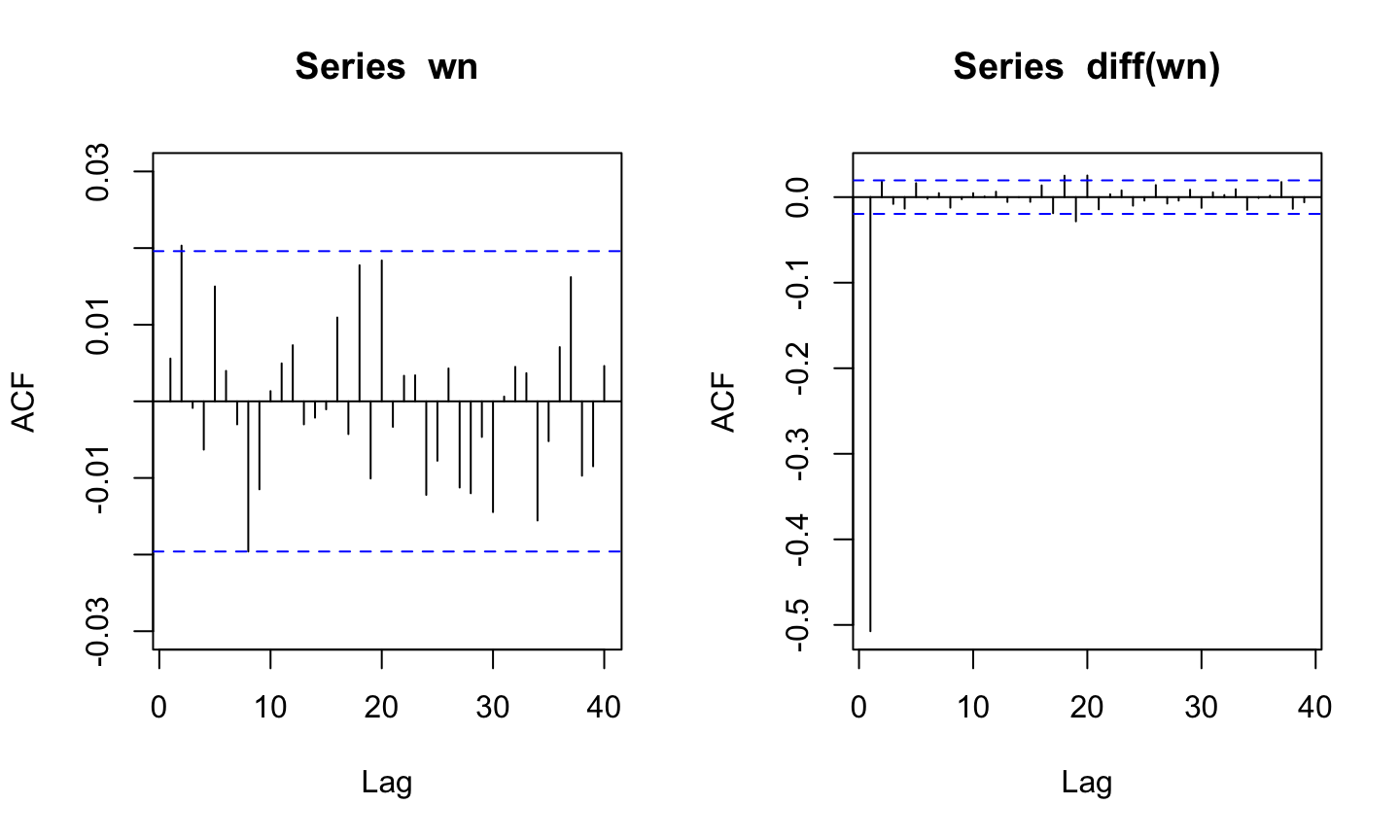## Case Study: Amazon (AMZN)

```# Amazon.com Stock Price, Daily, May 16, 1997 - Present
`plot(date, AMZN, type="l", col=2)`